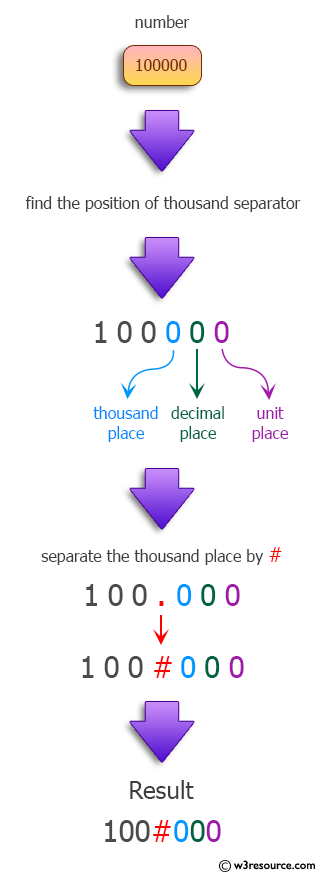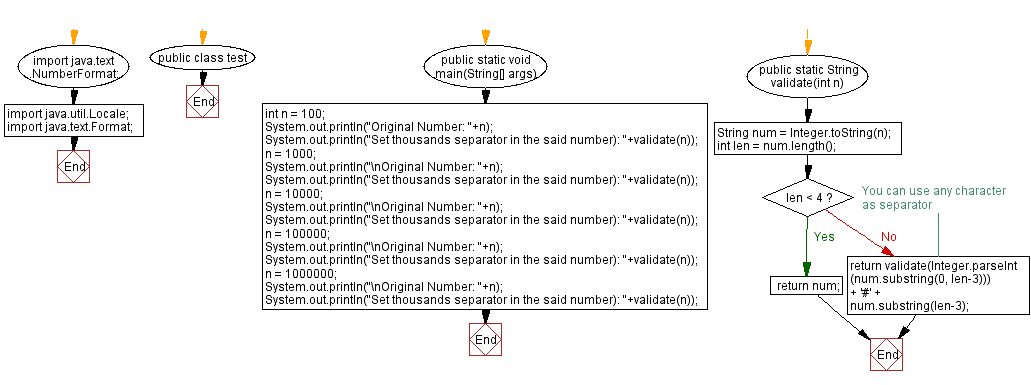﻿ Java: Set thousands separator in a given number# Java programming Exercises: Set thousands separator in the said number

## Java Regular Expression: Exercise-20 with Solution

Write a Java program that takes a number and set thousands separator in that number.

Sample Solution:

Java Code:

``````import java.text.NumberFormat;
import java.util.Locale;
import java.text.Format;

public class test {

public static void main(String[] args) {
int n = 100;
System.out.println("Original Number: "+n);
System.out.println("Set thousands separator in the said number): "+validate(n));
n = 1000;
System.out.println("\nOriginal Number: "+n);
System.out.println("Set thousands separator in the said number): "+validate(n));
n = 10000;
System.out.println("\nOriginal Number: "+n);
System.out.println("Set thousands separator in the said number): "+validate(n));
n = 100000;
System.out.println("\nOriginal Number: "+n);
System.out.println("Set thousands separator in the said number): "+validate(n));
n = 1000000;
System.out.println("\nOriginal Number: "+n);
System.out.println("Set thousands separator in the said number): "+validate(n));

}

public static String validate(int n) {
String num = Integer.toString(n);
int len = num.length();
if(len < 4) {
return num;
}
//You can use any character as separator
return validate(Integer.parseInt(num.substring(0, len-3))) + '#' + num.substring(len-3);
}
}
```
```

Sample Output:

```Original Number: 100
Set thousands separator in the said number): 100

Original Number: 1000
Set thousands separator in the said number): 1#000

Original Number: 10000
Set thousands separator in the said number): 10#000

Original Number: 100000
Set thousands separator in the said number): 100#000

Original Number: 1000000
Set thousands separator in the said number): 1#000#000
```

Pictorial Presentation:Flowchart :Java Code Editor:

Next: Remove all non-alphanumeric characters from a given string.

What is the difficulty level of this exercise?

Test your Programming skills with w3resource's quiz.

﻿

## Java: Tips of the Day

Split Java String by New Line

```String lines[] = string.split("\\r?\\n");
```

There's only really two newlines (UNIX and Windows) that you need to worry about.

Ref: https://bit.ly/3dHk7ip